# Kernel Regression using the Fast Fourier Transform

## 1. Setup

In a previous post it was shown how to speed up the computation of a kernel density using the Fast Fourier Transform. Conceptually a kernel density is not that far away from kernel regression, accordingly this post is will cover using the FFT to improve the computation of a kernel regression. A popular estimator for the regression functiongiven observed pointsis the Nadaraya–Watson estimator with kernel functionand bandwidth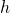:

(1)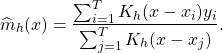The FFT requires a grid of with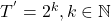design points. A meaningful choice in terms of asymptotic is. Thenwhich means thatandare asymptotically equivalent. In a first step we have to interpolateto fit to an equidistant grid with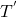points with

(2)Since we use an interpolation using an equidistant grid(1) becomes

(3)Let the discrete Fourier transform of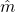be denoted by. The Fourier transform of (3) foris then using convolution and translation given by

(4)whereis the Fast Fourier transform of the interpolated data.can then be obtained by applying the inverse DFFT to (4). All these steps then involve onlyoperations instead ofoperations when computing (3) directly. Sorting, which may be required prior to the linear interpolation has a computational complexity ofwhich has the same order of magnitude than estimating the FFT based estimator itself and thus does not impact the computational complexity.

## 2. Asymptotic Considerations

An important question is if the interpolation decline the asymptotic properties of the estimator. Another way to describe the regression setup is fordesign points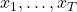in addition wlog for simplicity we requirethere are noisy observationssuch that

(5)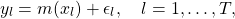for i.i.d. zero mean error terms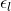with finite varianceand. Following , the Nadaraya–Watson estimator for an interior pointwe got a mean squared error of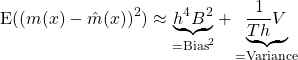while using a simple taylor extension, for neigbour pointsthe error introduced by the interpolation is given byPutting all together and using an appropriate bandwidth of order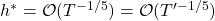using some straightforward calculations one can show that the interpolating error is dominated by the kernel regression error. A detailed proof and a extension allowing for multivariatecan be found in .

## 3. Python Code

To implement the method the fft function that comes with the numpy package was chosen. The numpy fft() function will return the approximation of the DFT from 0 to. Therefore fftshift() is needed to swap the output vector of the fft() right down the middle. So the output of fftshift(fft()) is then fromto.

import numpy as np
import matplotlib.pyplot as plt
import timeit
from scipy.interpolate import interp1d

# Simulation
T = 500
X = np.linspace(-5, 5, T)
Y= (X)**3 + np.random.normal(-0, 10, T)
plt.scatter(X, Y, c="blue")

plt.xlabel('sample')
plt.ylabel('X')

# Presetting
Tout = int( 2 ** np.floor(np.log(T)/np.log(2)) )
print(Tout)

# Interpolate Y
f = interp1d(X, Y)
grid = np.linspace(-5, 5, num=Tout)
Ynew=f(grid)

def epan_kernel(u, b):
u = u / b
return max(0, 1. / b * 3. / 4 * (1 - u ** 2))

# Estimation using FFT
start = timeit.default_timer()

t = grid
h= Tout**(-1/5)
kernel = [epan_kernel(x, h) for x in t]
kernel_ft = np.fft.fft(kernel)
func_tmp = kernel_ft.flatten() * np.fft.fft(Ynew)
m_fft = (max(X)-min(X))*np.fft.fftshift(np.fft.ifft(func_tmp).real)/Tout
plt.plot(t, m_fft)

stop = timeit.default_timer()
print('Time: ', stop - start)

plt.show()


### References

 {. Fan and {. Gijbels, Local polynomial modelling and its applications, London [u.a.]: Chapman & hall, 1996.
[Bibtex]
@book{Fan1996,
author = {Fan, {Jianqing} and Gijbels, {Irène}},
biburl = {http://www.bibsonomy.org/bibtex/23e163e04b09550b54a8067f0cbd97b7e/fbw_hannover},
intrahash = {3e163e04b09550b54a8067f0cbd97b7e},
isbn = {0412983214},
keywords = {Equations Mathematische_Statistik Polynomials Regression_analysis},
number = 66,
pagetotal = {XV, 341},
ppn_gvk = {19282144X},
publisher = {Chapman \& Hall},
series = {Monographs on statistics and applied probability series},
timestamp = {2009-08-21T10:31:17.000+0200},
title = {Local polynomial modelling and its applications},
url = {http://gso.gbv.de/DB=2.1/CMD?ACT=SRCHA&SRT=YOP&IKT=1016&TRM=ppn+19282144X&sourceid=fbw_bibsonomy},
year = 1996
}
 M. P. Wand, “Fast computation of multivariate kernel estimators,” Journal of computational and graphical statistics, vol. 3, iss. 4, p. 433–445, 1994.
[Bibtex]
@article{Wand1994,
ISSN = {10618600},
URL = {http://www.jstor.org/stable/1390904},
abstract = {Multivariate extensions of binning techniques for fast computation of kernel estimators are described and examined. Several questions arising from this multivariate extension are addressed. The choice of binning rule is discussed, and it is demonstrated that linear binning leads to substantial accuracy improvements over simple binning. An investigation into the most appropriate means of computing the multivariate discrete convolutions required for binned kernel estimators is also given. The results of an empirical study indicate that, in multivariate settings, the fast Fourier transform offers considerable time savings compared to direct calculation of convolutions.},
author = {M. P. Wand},
journal = {Journal of Computational and Graphical Statistics},
number = {4},
pages = {433--445},
publisher = {[American Statistical Association, Taylor & Francis, Ltd., Institute of Mathematical Statistics, Interface Foundation of America]},
title = {Fast Computation of Multivariate Kernel Estimators},
volume = {3},
year = {1994}
}

This site uses Akismet to reduce spam. Learn how your comment data is processed.